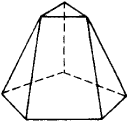# Prismatoid

Also found in: Dictionary, Thesaurus, Medical, Wikipedia.

## prismatoid

[′priz·mə‚tȯid]
(mathematics)
A polyhedron whose vertices all are in one or the other of two parallel planes.

## Prismatoid

a polyhedron in which two faces (the bases of the prismatoid) lie in parallel planes and the remaining faces areFigure 1

triangles or trapezoids, where one side of each triangle and two sides of each trapezoid are edges of the bases (see Figure 1). The volume of a prismatoid is equal to (h/6) (S + S′ + 4S″), where h is the perpendicular distance between the bases, S and S′ are the areas of the bases, and S″ is the area of a plane section midway between the two bases.

Site: Follow: Share:
Open / Close